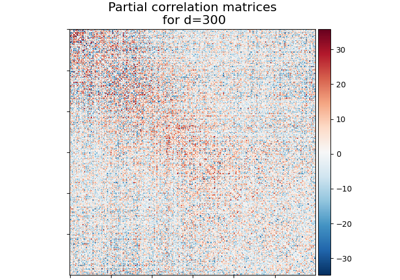Note

This page is a reference documentation. It only explains the function signature, and not how to use it. Please refer to the user guide for the big picture.

# nilearn.datasets.fetch_megatrawls_netmats#

nilearn.datasets.fetch_megatrawls_netmats(dimensionality=100, timeseries='eigen_regression', matrices='partial_correlation', data_dir=None, resume=True, verbose=1)[source]#

This data can be used to predict relationships between imaging data and non-imaging behavioural measures such as age, sex, education, etc. The network matrices are estimated from functional connectivity datasets of 461 subjects. Full technical details in references.

Parameters:
dimensionalityint, optional

Valid inputs are 25, 50, 100, 200, 300. By default, network matrices estimated using Group ICA brain parcellations of 100 components/dimensions will be returned. Default=100.

timeseriesstr, optional

Valid inputs are ‘multiple_spatial_regression’ or ‘eigen_regression’. By default ‘eigen_regression’, matrices estimated using first principal eigen component timeseries signals extracted from each subject data parcellations will be returned. Otherwise, ‘multiple_spatial_regression’ matrices estimated using spatial regressor based timeseries signals extracted from each subject data parcellations will be returned. Default=’eigen_regression’.

matricesstr, optional

Valid inputs are ‘full_correlation’ or ‘partial_correlation’. By default, partial correlation matrices will be returned otherwise if selected full correlation matrices will be returned. Default=’partial_correlation’.

data_dir`pathlib.Path` or `str`, optional

resume`bool`, default=True

verbose`int`, default=1

Verbosity level (0 means no message).

Returns:
dataBunch

Dictionary-like object, the attributes are :

• ‘dimensions’: int, consists of given input in dimensions.

• ‘timeseries’: str, consists of given input in timeseries method.

• ‘matrices’: str, consists of given type of specific matrices.

• ‘correlation_matrices’: ndarray, consists of correlation matrices based on given type of matrices. Array size will depend on given dimensions (n, n).

• ‘description’: data description

Notes

See description for terms & conditions on data usage.

References

## Examples using `nilearn.datasets.fetch_megatrawls_netmats`#Visualizing Megatrawls Network Matrices from Human Connectome Project

Visualizing Megatrawls Network Matrices from Human Connectome Project